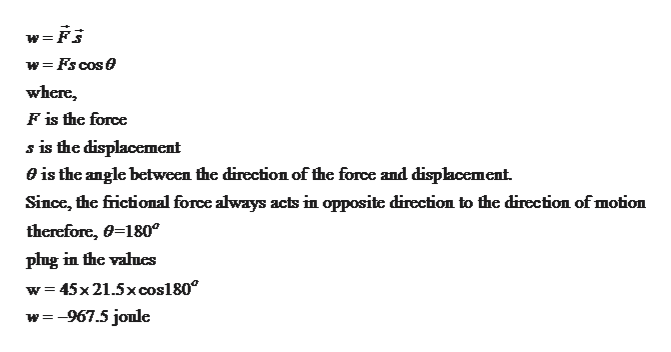# A shopper pushes a grocery cart for a distance 21.5 m at constant speed on level ground, against a 45 N frictional force. He pushes in a direction 27.5° below the horizontal.a.) What is the work done on the cart by friction, in joules?b.)What is the work done on the cart by the gravitational force, in joules? c.) What is the work done on the cart by the shopper, in joules? d.) Find the magnitude of the force, in newtons, that the shopper exerts on the cart. e.) What is the total work done on the cart, in joules?

Question
13 views

A shopper pushes a grocery cart for a distance 21.5 m at constant speed on level ground, against a 45 N frictional force. He pushes in a direction 27.5° below the horizontal.

a.) What is the work done on the cart by friction, in joules?

b.)What is the work done on the cart by the gravitational force, in joules?

c.) What is the work done on the cart by the shopper, in joules?

d.) Find the magnitude of the force, in newtons, that the shopper exerts on the cart.

e.) What is the total work done on the cart, in joules?

check_circle

Step 1

Since we only answer up to 3 sub-parts, we’ll answer the first 3. Please resubmit the question and specify the other subparts (up to 3) you’d like answered.

Step 2

Given:

Distance travelled = 21.5 m

Frictional force = 45 N

Angle with respect to horizontal = 27.5

Step 3

Part (a): Work done on the car...help_outlineImage Transcriptionclosew=F5 = Fscose where F is the force s is the displacement 0is the angle between the direction of the force and displacement. Since, the frictional force always acts in opposite direction to the dircction of motion therefore, 0-180 plng in the vaues w 45x21.5x cos180 w967.5 jonle fullscreen

### Want to see the full answer?

See Solution

#### Want to see this answer and more?

Solutions are written by subject experts who are available 24/7. Questions are typically answered within 1 hour.*

See Solution
*Response times may vary by subject and question.
Tagged in

### Work,Power and Energy